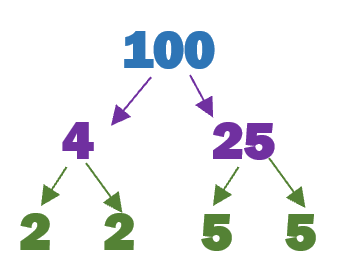Home > Math Topics > Integers >

## Prime Factorization and Factor Trees

#### Grade 6 Numbers - 6.NS.B.4

What is Prime Factorization? Factors of a number mean the parts that will compose a whole number. Factors of a number mean the numbers when multiplied together form the original number. for instance, Factors of 6 could be 2 and 3, because the multiplication of these numbers will make the original number 6. Other factors of 6 are 1,2 3, and 6. These all when multiplied with each other give us the same result, i.e., 6. 1 x 6=6, 2 x 3= 6, 3 x 2 = 6, 6x 1= 6 PRIME FACTORS - Numbers that are divisible only by themselves are known as prime factors. Some of the prime numbers include 2, 5, 17, 19. These numbers are found only in their own times tables and are divisible by 1 and by themselves. PRIME FACTORIZATION - Factorization of a number means to break it down into smaller parts. To do prime factorization of a number, we break down the numbers into its prime factors. Prime factorization of a number starts with using a factor tree. A factor tree is a diagram used for breaking down the numbers into factors to a point where only its prime factors remain. To do prime factorization of a number, we first divide the prime numbers only. Let's take 24 as an example. For most even numbers, we use 2 as the first prime factor. Since 24 is an even number, factoring out 2, will give us, 2 x 12. Next, 12 is also an even number, so we will factor out 2 again. This will give us 2x 2x 6. Again, 6 is an even number. We factor out another 2, which will leave us with 2x 2 x2 x3. Observe that these all are prime numbers. The point where you get all the prime number, your prime factorization is complete These worksheets and lessons will help students understand how to process prime factorization and create factor trees.

### Printable Worksheets And Lessons#### Homework Sheets

We get really familiar with using Factor Trees.

• Homework 1 - Complete the factor chart.
• Homework 2 - We first look at all of the factors of the value.
• Homework 3 - We also notice that one of the factors must be able to be broken down twice. We have several choices to choose from.

#### Practice Worksheets

These sheets advance the skills a bit more and use larger numbers.

• Practice 1 - This one is slightly tricky because one of the factors has to be able to be factored one step further.
• Practice 2 - All of the factors would work except for 2 or 3. Choose one to work with.
• Practice 3 - See where they fall within the chart and move on from there.

#### Math Skill Quizzes

I tried to limit the factor charts to three steps.

• Quiz 1 - These are pretty easy to get you going.
• Quiz 2 - The odd numbers always throw kids for a loop.
• Quiz 3 - Where do we go with this.

### What Are Factor Trees?

Just like a family tree displays all the descendants and people that came before them, factor trees display all the factors that came to create values before it. As we remember factors are numbers that you can multiply together to get a fixed or specified product. Factor trees are common visual diagrams that are used to find the prime factors of natural numbers. The value that you are exploring must be greater than one. As we further break down values, we can also create a pathway to determine the prime factorization of the original value. Look at the factor tree of 100 below. We know that when we multiply 4 and 25 the product forms 100. This serves as our first layer of the tree. Now we further break down 4 and 25 into their respective factors on the secondary layer (2 x 2) and (5 x 5). You can no longer factor the remaining values, so your tree is complete. As you can see this tool is great for quickly determining and setting up prime factorization of numbers that you come across.